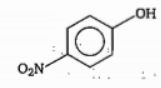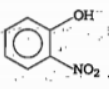Which of the following compound has the smallest bond angle (X$—$A$—$Z) in each series respectively?

(A)              $OSB{r}_{2}$

(B) $SbC{I}_{3}$     $SbB{r}_{3}$              $Sb{I}_{3}$

(C) $P{I}_{3}$         $As{I}_{3}$                  $Sb{I}_{3}$

Options are as follows:

(a)     (b)

(c)         (d)

Concept Questions :-

Hybridisation
High Yielding Test Series + Question Bank - NEET 2020

Difficulty Level:

Which species has the same shape as $N{H}_{3}$

(a) $S{{O}_{3}}^{2-}$

(b) $C{{O}_{3}}^{2-}$

(c) $N{{O}_{3}}^{-}$

(d) $S{O}_{3}$

Concept Questions :-

Hybridisation
High Yielding Test Series + Question Bank - NEET 2020

Difficulty Level:

According to VSEPR theory in, which species do all the atoms lie in the same plane?

1. $C{{H}_{3}}^{+}$                                   2. $C{{H}_{3}}^{-}$

(a) 1 only                                (b) 2 only

(c) both 1 and 2                      (d) neither 1 nor 2

Concept Questions :-

Hybridisation
High Yielding Test Series + Question Bank - NEET 2020

Difficulty Level:

Idetify the correct sequence of increasing number of $\mathrm{\pi }$ bonds in the structure of the following molecules :

(I) ${H}_{2}{S}_{2}{O}_{6}$     (II) ${H}_{2}{S}_{2}{O}_{3}$   (III)  ${H}_{2}{S}_{2}{O}_{5}$

(a) I II and III         (b) II I and III

(c) II III and I         (d) I III and II

High Yielding Test Series + Question Bank - NEET 2020

Difficulty Level:

The state of hybridisation of central atom in dimer of $B{H}_{3}$ and $Be{H}_{2}$ is:

(a) $s{p}^{2},s{p}^{2}$    (b) $s{p}^{3},s{p}^{2}$  (c) $s{p}^{3},s{p}^{3}$  (d) $s{p}^{2},s{p}^{3}$

High Yielding Test Series + Question Bank - NEET 2020

Difficulty Level:

Which of the following species/molecules does not have same number of bond pairs and lone pairs?

(a) $OC{N}^{-}$

(b) ${H}_{2}O$

(c) ${C}_{2}{H}_{2}C{l}_{2}$

(d) ${O}_{3}$

High Yielding Test Series + Question Bank - NEET 2020

Difficulty Level:

${O}_{2}{F}_{2}$ is an unstable yellow orange solid and ${H}_{2}{O}_{2}$ is a colourless liquid both have O$—$O bond and

O$—$O bond length in  ${H}_{2}{O}_{2}$ and ${O}_{2}{F}_{2}$ respectively is:

(a) 1.22 A, 1.48               (b) 1.48 A, 1.22A

(c) 1.22 A, 1.22A             (d) 1.48 A, 1.48A

Concept Questions :-

Resonance
High Yielding Test Series + Question Bank - NEET 2020

Difficulty Level:

The bond length of the S$—$O bond in maximum in which of the following compounds?

(a)

(b) $SOB{r}_{2}$

(c)$SO{F}_{2}$

(d) All have the same length

Concept Questions :-

Covalent Bonding
High Yielding Test Series + Question Bank - NEET 2020

Difficulty Level:

Out of the two compounds shown below, the vapour pressure of B at a particular tempreture is expected to be :and(A)                                  (B)

(a) Highr than that of A

(b) Lower than that of A

(c) same as that of A

(d) can be higher or lower depending upon the size of the vessel

Concept Questions :-

Hydrogen Bonding
High Yielding Test Series + Question Bank - NEET 2020

Difficulty Level:

solubillity of alkali metal fluorides increases down the group select correct explanation for given statement :

(a) Hydration energy increase and lattice energy decrease down the group

(b) both energy decrease down the group but decrease in hyderation energy is rapid

(c) both energy decrease down the group but decrease in lattice energy is rapid

(d) both energy decrease down the group but increase in hyderation energy is rapid

Concept Questions :-

Lattice/Hydration Energy